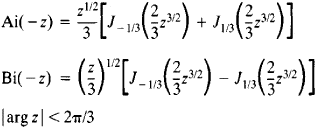Airy Function

Also found in: Wikipedia.

Airy function

[¦er·ē ¦fəŋk·shən]
(mathematics)
Either of the solutions of the Airy differential equation.
McGraw-Hill Dictionary of Scientific & Technical Terms, 6E, Copyright © 2003 by The McGraw-Hill Companies, Inc.
The following article is from The Great Soviet Encyclopedia (1979). It might be outdated or ideologically biased.

Airy Function

either of the functions Ai(z) and Bi(z), which are solutions of the second-order differential equation

W″zW = 0

where z is the independent variable.

The Airy functions of the argument (–z) may be expressed in terms of Bessel functions of order v = ±⅓:The asymptotic representations for large |z| areAiry functions play an important role in the theory of asymptotic representations of various special functions; they have diverse applications in mathematical physics—for example, in the theory of the diffraction of radio waves at the earth’s surface. Airy functions were studied by J. R. Airy in 1911.

REFERENCES

Lebedev, N. N. Spetsial’nye funktsii i ikh prilozheniia. 2nd ed. Moscow-Leningrad, 1963.
References in periodicals archive ?
At the same time, a considerable number of frontier and interdisciplinary applications, such as optical microparticles manipulation , formation of Airy beam lasers , generation of optical bullets , routing of surface plasmon polaritons , generation of curved plasma channels , and creating curved electron beams without any external field , have been took advantage of the Airy function and Airy beam technology.
where Ai[??] denotes the Airy function, [x.sub.0] and [y.sub.0] are beams horizontal size and vertical size, respectively, [a.sub.0] and [b.sub.0] represent exponential modulation factors, and E[??] is electric field intensity on incident surface.
The Airy function v([zeta] - [nu]), which represents the incident plane wave in (6), is replaced with the Whittaker function [M.sub.it,n](-i[chi][tau]), while the Airy function [w.sub.1]([zeta] - [nu]) which represents the secondary wave outgoing from the surface is replaced with the Whittaker function [W.sub.it,n](-i[chi][tau]).
An argument of Airy function equation (31) can be further expanded, taking into account large values of [mu] ~ [[mu].sub.0] [much greater than] 1:
where [x.sub.k] is the kth zero of the Airy function and the quantities [gamma] and [beta] are two scaling and fitting parameters, respectively, that are used to take into account both the effects of anisotropy ([gamma] parameter) and the other displacement effects ([beta] parameter).
The specific form of the wave packet can be finally expressed by using some special functions, slay, in terms of oscillating exponentials, Airy function, Fresnel integral, Pearcey- type integral, etc.
Note that the allowed values of energy E implicitly enter in (6) in the form of the Airy function arguments in (5).
where Ai([rho](x)) is the Airy function and its complement is Bi([rho](x)) .
Similar, but thinner shapes, also part of the Airy function, extended parallel to the main parabola, the team reports in the Nov.
On the other hand, if I = 3 the integral is described asymptotically by the Airy function. In this case the integral in (4) has typically an asymptotic series expansion which is a linear combination of terms of order ([[parallel]([n.sub.0], n)[parallel].sup.-l-1/3)] l[greater than or equal to]0 and also of order ([[parallel] ([n.sub.0],n)[parallel].sup.-l-2/3)]l[greater than equal to] 0.
As the 3j, 6j, 9j (angular momenta) and Airy functions chapters were no more dense than the above two, I must have started with the worst!
Site: Follow: Share:
Open / Close